/

### Showing that VaR is not coherent for exponentially distributed loss variables

Please bear in mind that in general a multivariate distribution each of the marginal of which is exponentially distributed is not necessarily a member of the exponential family of (multivariate) distributions.

The simplest case to consider is where two loss variables come from the same exponential distribution with parameter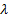. The probability density function of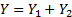where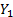and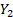are independent random variables each coming from an exponential distribution with parameteris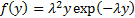.

The VaR at confidence level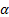for,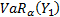is the same as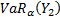and is the value of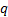for which: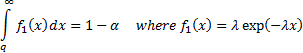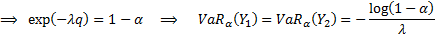The VaR at the same confidence level for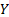is  the value offor which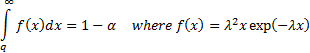Integrating by parts we have: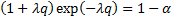The exponential distribution (with independent variables) is not elliptical so VaR’s for it shouldn’t be coherent. More specifically it should be possible to identify a suitablefor which VaR is not sub-additive, i.e. for which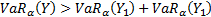, as sub-additivity is one of the axioms required for coherence. Although there is no certainty that this is the case it is likely that such a situation will arise either whereis close to zero or whenis close to 1. The case whereis close to 1 does not look promising as the behaviour of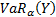will tend to be dominated by the exponential term. So instead let us consider the case where whenis close to 0. We then have for: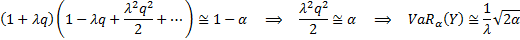However in these circumstances: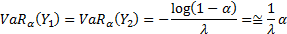So ifis small enough we will have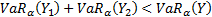as desired to prove the conjecture.